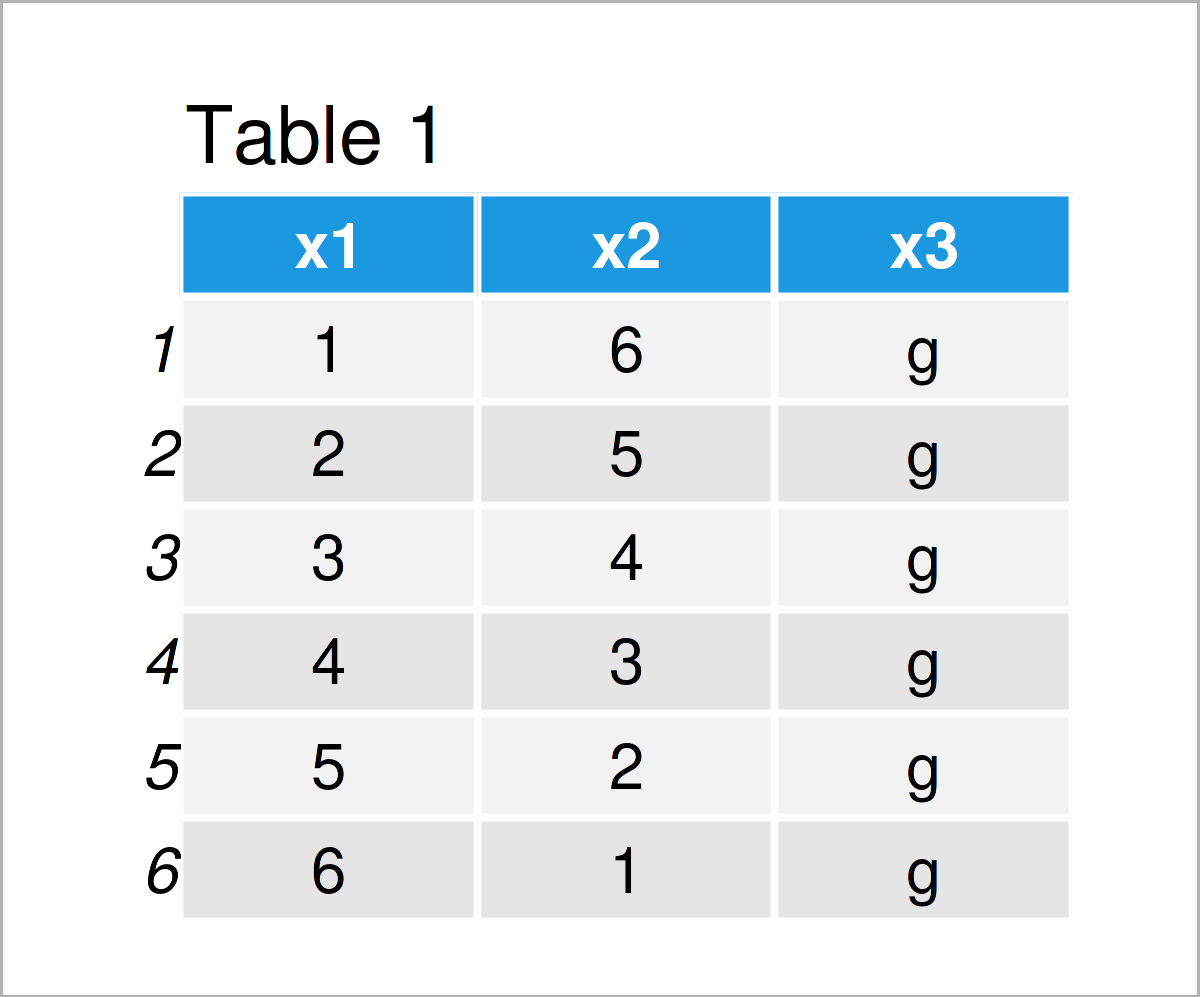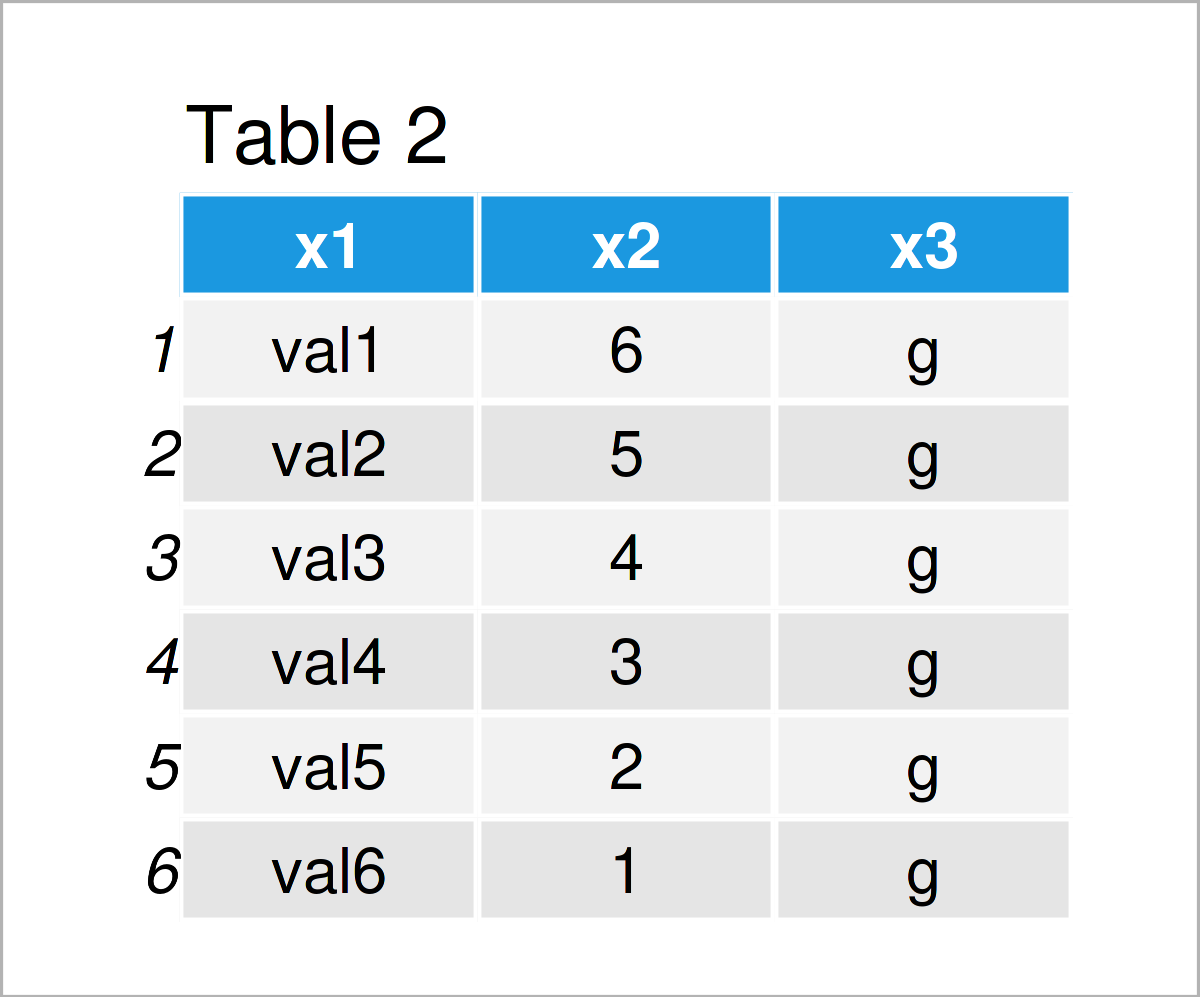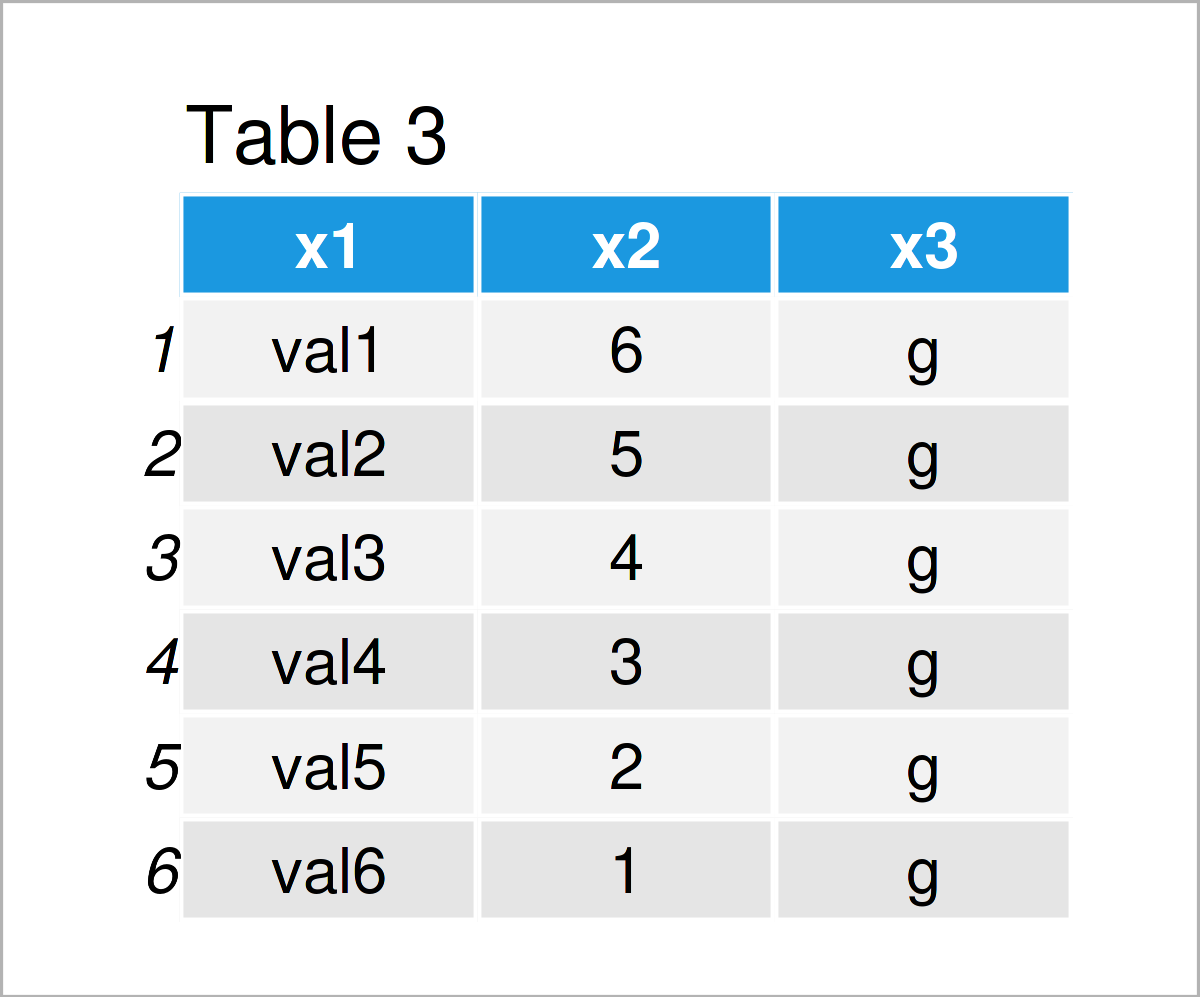# Add Characters to Numeric Data Frame Column in R (2 Examples)

This tutorial illustrates how to append characters in front of a numeric data frame variable in the R programming language.

The content looks as follows:

Let’s dive into it…

## Creation of Example Data

First, we’ll need to construct some data that we can use in the examples below:

```data <- data.frame(x1 = 1:6,                     # Create example data frame
x2 = 6:1,
x3 = "g")
data                                             # Print example data frame```As you can see based on Table 1, our example data is a data frame consisting of six rows and three columns. The variables x1 and x2 have the integer class and the variable x3 is a character.

## Example 1: Append Characters in Front of Numeric Values in Data Frame Column Using paste0() Function

This example explains how to add characters in front of the numbers of a data frame column (in this case with the integer class, but the same would be possible for a numeric column).

To accomplish this, we can apply the paste0 function as shown below:

```data_new1 <- data                                # Duplicate data frame
data_new1\$x1 <- paste0("val", data_new1\$x1)      # Apply paste0 function
data_new1                                        # Print new data frame```As shown in Table 2, we have created a new data frame called data_new1 by executing the previous R syntax. In this data frame, we have added the prefix “val” to the first column x1.

Note that the syntax of this Example has converted our integer column to the character class.

## Example 2: Append Characters in Front of Numeric Values in Data Frame Column Using sub() Function

This example demonstrates how to add characters to the numbers of a data frame column using the sub function.

Consider the R programming code below:

```data_new2 <- data                                # Duplicate data frame
data_new2\$x1 <- sub("^", "val", data_new2\$x1)    # Apply sub function
data_new2                                        # Print new data frame```After running the previous R programming syntax the new data frame called data_new2 shown in Table 3 has been created.

This data frame contains exactly the same modifications as the data frame created in Example 1. However, this time we have applied the sub function instead of the paste0 function.

## Video, Further Resources & Summary

I have recently released a video on my YouTube channel, which explains the R code of this article. You can find the video below.

In addition, you could have a look at the related tutorials on my website. I have released numerous tutorials already:

This article has shown how to add characters at the beginning of a numeric data frame column in R programming. If you have further questions, please let me know in the comments section below.

Subscribe to the Statistics Globe Newsletter